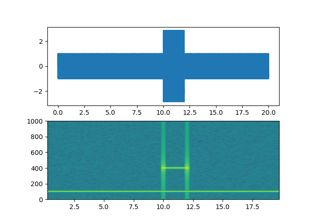# matplotlib.axes.Axes.specgram#

Axes.specgram(x, NFFT=None, Fs=None, Fc=None, detrend=None, window=None, noverlap=None, cmap=None, xextent=None, pad_to=None, sides=None, scale_by_freq=None, mode=None, scale=None, vmin=None, vmax=None, *, data=None, **kwargs)[source]#

Plot a spectrogram.

Compute and plot a spectrogram of data in x. Data are split into NFFT length segments and the spectrum of each section is computed. The windowing function window is applied to each segment, and the amount of overlap of each segment is specified with noverlap. The spectrogram is plotted as a colormap (using imshow).

Parameters:
x1-D array or sequence

Array or sequence containing the data.

Fsfloat, default: 2

The sampling frequency (samples per time unit). It is used to calculate the Fourier frequencies, freqs, in cycles per time unit.

windowcallable or ndarray, default: `window_hanning`

A function or a vector of length NFFT. To create window vectors see `window_hanning`, `window_none`, `numpy.blackman`, `numpy.hamming`, `numpy.bartlett`, `scipy.signal`, `scipy.signal.get_window`, etc. If a function is passed as the argument, it must take a data segment as an argument and return the windowed version of the segment.

sides{'default', 'onesided', 'twosided'}, optional

Which sides of the spectrum to return. 'default' is one-sided for real data and two-sided for complex data. 'onesided' forces the return of a one-sided spectrum, while 'twosided' forces two-sided.

The number of points to which the data segment is padded when performing the FFT. This can be different from NFFT, which specifies the number of data points used. While not increasing the actual resolution of the spectrum (the minimum distance between resolvable peaks), this can give more points in the plot, allowing for more detail. This corresponds to the n parameter in the call to `fft`. The default is None, which sets pad_to equal to NFFT

NFFTint, default: 256

The number of data points used in each block for the FFT. A power 2 is most efficient. This should NOT be used to get zero padding, or the scaling of the result will be incorrect; use pad_to for this instead.

detrend{'none', 'mean', 'linear'} or callable, default: 'none'

The function applied to each segment before fft-ing, designed to remove the mean or linear trend. Unlike in MATLAB, where the detrend parameter is a vector, in Matplotlib it is a function. The `mlab` module defines `detrend_none`, `detrend_mean`, and `detrend_linear`, but you can use a custom function as well. You can also use a string to choose one of the functions: 'none' calls `detrend_none`. 'mean' calls `detrend_mean`. 'linear' calls `detrend_linear`.

scale_by_freqbool, default: True

Whether the resulting density values should be scaled by the scaling frequency, which gives density in units of 1/Hz. This allows for integration over the returned frequency values. The default is True for MATLAB compatibility.

mode{'default', 'psd', 'magnitude', 'angle', 'phase'}

What sort of spectrum to use. Default is 'psd', which takes the power spectral density. 'magnitude' returns the magnitude spectrum. 'angle' returns the phase spectrum without unwrapping. 'phase' returns the phase spectrum with unwrapping.

noverlapint, default: 128

The number of points of overlap between blocks.

scale{'default', 'linear', 'dB'}

The scaling of the values in the spec. 'linear' is no scaling. 'dB' returns the values in dB scale. When mode is 'psd', this is dB power (10 * log10). Otherwise this is dB amplitude (20 * log10). 'default' is 'dB' if mode is 'psd' or 'magnitude' and 'linear' otherwise. This must be 'linear' if mode is 'angle' or 'phase'.

Fcint, default: 0

The center frequency of x, which offsets the x extents of the plot to reflect the frequency range used when a signal is acquired and then filtered and downsampled to baseband.

cmap`Colormap`, default: `rcParams["image.cmap"]` (default: `'viridis'`)
xextentNone or (xmin, xmax)

The image extent along the x-axis. The default sets xmin to the left border of the first bin (spectrum column) and xmax to the right border of the last bin. Note that for noverlap>0 the width of the bins is smaller than those of the segments.

dataindexable object, optional

If given, the following parameters also accept a string `s`, which is interpreted as `data[s]` (unless this raises an exception):

x

**kwargs

Additional keyword arguments are passed on to `imshow` which makes the specgram image. The origin keyword argument is not supported.

Returns:
spectrum2D array

Columns are the periodograms of successive segments.

freqs1-D array

The frequencies corresponding to the rows in spectrum.

t1-D array

The times corresponding to midpoints of segments (i.e., the columns in spectrum).

im`AxesImage`

The image created by imshow containing the spectrogram.

`psd`

Differs in the default overlap; in returning the mean of the segment periodograms; in not returning times; and in generating a line plot instead of colormap.

`magnitude_spectrum`

A single spectrum, similar to having a single segment when mode is 'magnitude'. Plots a line instead of a colormap.

`angle_spectrum`

A single spectrum, similar to having a single segment when mode is 'angle'. Plots a line instead of a colormap.

`phase_spectrum`

A single spectrum, similar to having a single segment when mode is 'phase'. Plots a line instead of a colormap.

Notes

The parameters detrend and scale_by_freq do only apply when mode is set to 'psd'.

## Examples using `matplotlib.axes.Axes.specgram`#Spectrogram Demo

Spectrogram Demo